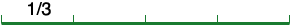SEARCH HOMEMath Central Quandaries & QueriesQuestion from larry, a parent: Hello, My math question is. If a third is a fourth of a number, what is the number? Thank you,Hi Larry,

Think of the unknown number as the length of a line segment. What you know is that the length of one fourth of the segment is one third.Thus the lengths of the other three fourths of the line segment are also one third so the line segment has length

1/3 + 1/3 + 1/3 + 1/3 = 4/3.

PennyMath Central is supported by the University of Regina and The Pacific Institute for the Mathematical Sciences.